# Thiele hard sphere equation of stateThis article is a 'stub' page, it has no, or next to no, content. It is here at the moment to help form part of the structure of SklogWiki. If you add sufficient material to this article then please remove the {{Stub-general}} template from this page.

The Thiele hard sphere equation of state is an equation of state for a hard sphere fluid developed by Thiele in 1963 . The equation provides a better approximation of the repulsive forces between molecules than the Van der Waals repulsive term. The equation is given below: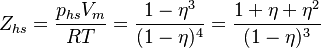$Z_{hs} = \frac{p_{hs}V_m}{RT} = \frac{1 - \eta^3}{(1-\eta)^4} = \frac{1 + \eta + \eta^2}{(1-\eta)^3}$,

where: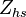$Z_{hs}$ is the compressibility factor of the hard sphere fluid;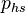$p_{hs}$ is the pressure of the fluid;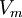$V_m$ is the molar volume of the fluid;$T$ is the absolute temperature of the fluid;$R$ is the gas constant; and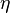$\eta$ is the packing fraction of the fluid.

In terms of accuracy, the Thiele equation is superseded by the Carnahan-Starling equation of state